## Protecting features using vertex locks and weights

Disclaimer: The code in this post is written on version 10.0.4600 of Simplygon. Should you encounter this post running a different version, some of the API calls might differ. However, the core concepts should still remain valid.

## Overview

In this blog post we will go through different ways of protecting features in your assets. We will use a character face to demonstrate. Small differences in character faces are easily detectable and can be percieved as errors. The goal in our example is to protect the areas around the eyes, nose and mouth to ensure that the face is kept good looking even at lower reduction rates.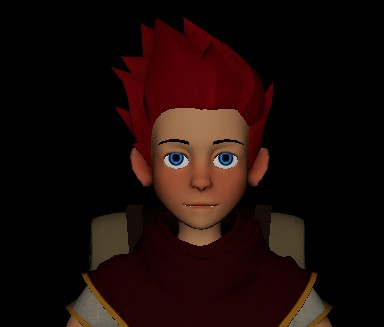The example asset

## Vertex weights

One way of protecting these features is by painting vertex weights to tell Simplygon where to keep more geometry (or vice versa). By adding a color set to the character and painting the areas we want to protect we can then tell the reducer to respect those weights.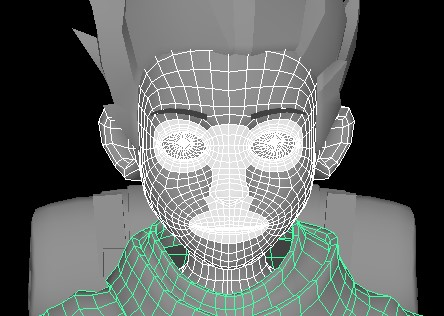Vertex weights protecting the areas around the eyes, nose and mouth.

The asset we are using is built up by quads, which means that we can use the quad reducer to reduce it. Typically you wouldn't need to protect the face topology like this with the quad reducer as it removes whole quad loops, as opposed to removing individual triangles as the regular reducer does. Keep in mind though that the vertex weights are not protecting the individual quads in the quad reducer, but rather instructs the reducer to take extra care with the quad strips passing through that quad.

Here is how you can set up the quad reducer to take the weights into account. In our case we named the color set weights, but it could be called whatever you choose.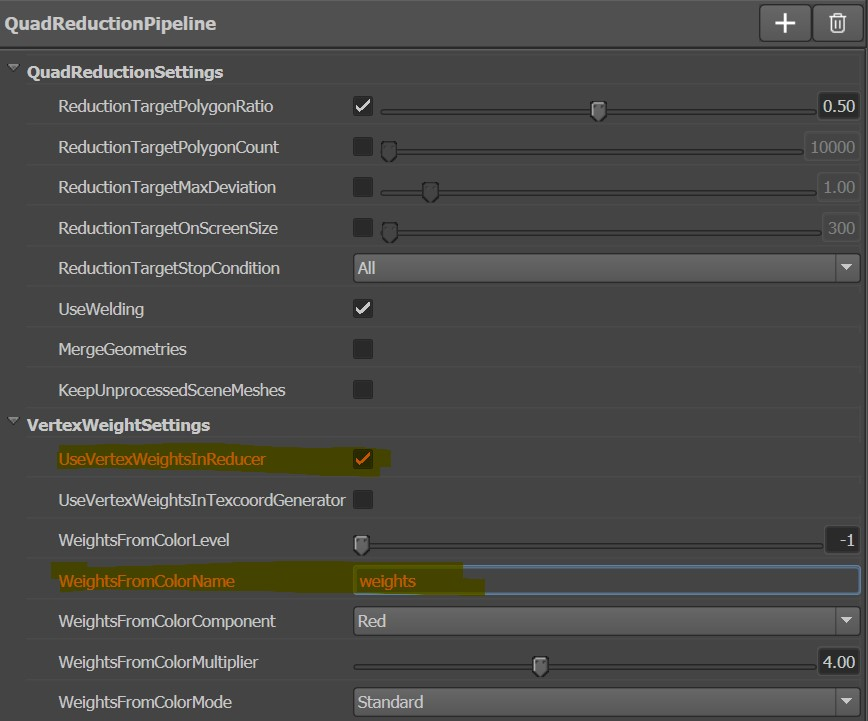The WeightsFromColorMultiplier is worth looking closer at. The multiplier tells Simplygon how much weight to put behind the weights. It might look odd to have another layer to take into account when there is a grayscale at your disposal in the color range (where white means keep and black means take away). This might be the true in the case of a single asset, but this knob gives you the option to apply change the weighting factor across a large set of assets. It could be valuable tool as you're tuning in the optimization settings for your characters, for example.

Here is the face after running it through the quad reducer: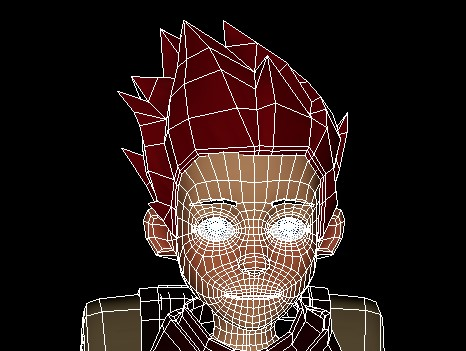The triangle reducer offers the same way of protecting areas, and it that case it also makes more sense as the wrong triangle removed around the mouth, for example, could really change the appearance of the face. The vertex weight protection also becomes more apparent when looking at the results for the triangle reducer.Keep in mind that vertex weighting is relative and not the same thing as locking the vertices. Simplygon will always meet the optimization goals, meaning that if you tell Simplygon to remove 50% of the polygons and paint the half the model white it would almost be the equivalent of deleting the other half of the model.

## Vertex locks

While vertex weights are really useful when you need to "soft lock" vertices, there are cases where you want to ensure that certain vertices/areas are kept no matter what. In these cases you can provide Simplygon a selection set containing the vertices that you want to lock.

There are two limitations with the vertex selection sets:

• They are not yet implemented (10.0.4600) in the Quad Reducer.
• You cannot use them through the regular UI settings, but need to provided either through the API or the Max and Maya command.

In 3DS Max you need to use the commmand sgsdk_SetLockSelectedVertices to lock vertices as you launch Simplygon.

In Maya you need to provide the selected vertices through the LockSetVertices(lsv) flag.

### Using vertex locks through Maya

Let's start by selecting a set of vertices that we want to protec and create a selection set for them.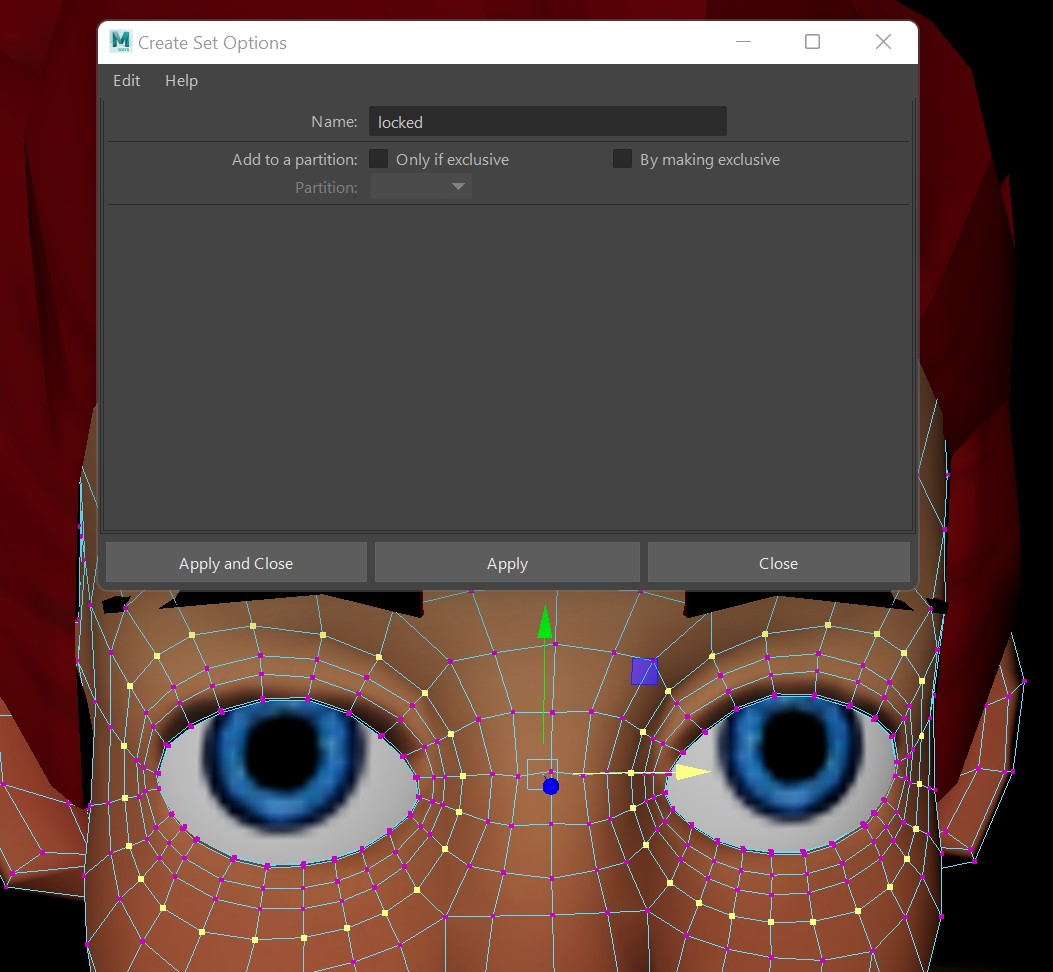If we want to run, for example, a 50% reduction on this character that keeps these vertices locks we can create a reduction pipeline in the UI and save that somewhere on the disk.Now that we have all this in place we can just run this command:

Simplygon -lsv "locked" -sf "path to settings file"


Here is what the character looks like after the optimization process with locked verts.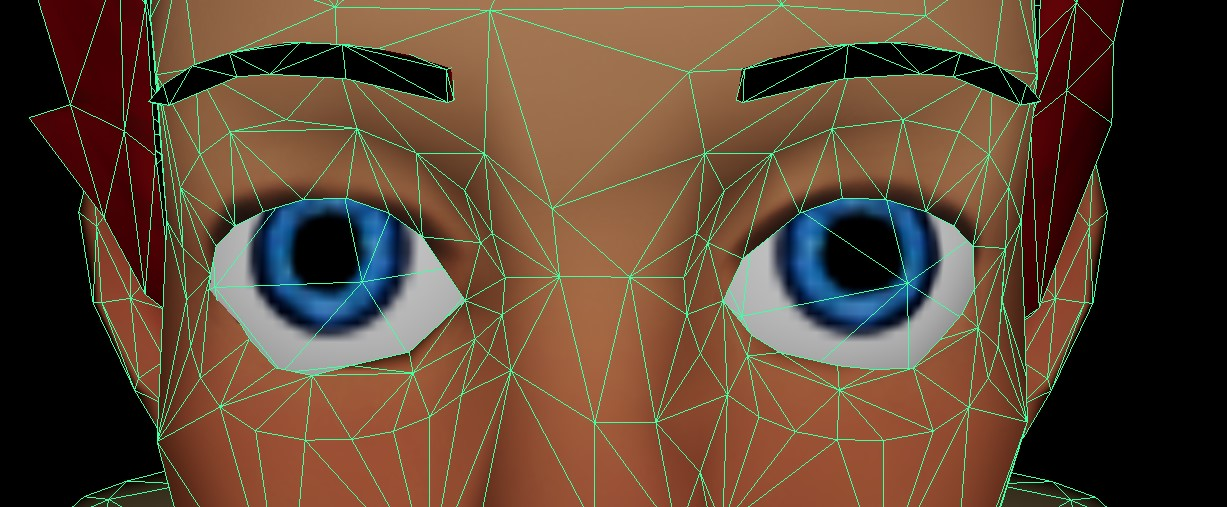## Using vertex weights and locks through script

If you are looking to create an automatic optimization pipeline with the abilities mentioned above, you can use this in script as well.

This function would can take an asset file with a color channel named weights and reduce it with the weights in mind.

weights_channel =  "weights"

def reduce_with_weights(sg, asset_file, output_file):
scene_importer = sg.CreateSceneImporter()
scene_importer.SetImportFilePath(asset_file)
if scene_importer.Run() == Simplygon.EErrorCodes_NoError:
scene = scene_importer.GetScene()
reduction_pipeline = sg.CreateReductionPipeline()
reduction_settings = reduction_pipeline.GetReductionSettings()
reduction_settings.SetReductionTargetTriangleRatio(0.5)

weight_settings = reduction_pipeline.GetVertexWeightSettings()
# Make sure that the reducer uses the weights
weight_settings.SetUseVertexWeightsInReducer(True)
# Specify the weight channel
weight_settings.SetWeightsFromColorName(weights_channel)
# Set how much the weights should influence the reducer. 10 is max.
weight_settings.SetWeightsFromColorMultiplier(10)

reduction_pipeline.RunScene(scene, Simplygon.EPipelineRunMode_RunInThisProcess)

scene_exporter = sg.CreateSceneExporter()
scene_exporter.SetExportFilePath(output_file)
scene_exporter.SetScene(scene)
scene_exporter.Run()


The above function is pretty straight forward, just standard Simplygon settings. When it comes to locks it becomes a little more involved. Selection sets is not a first class citizen of most file formats, which means that the information of which vertices to lock needs to be added afterwards. This can be achieved by transfering vertex colors into locks.

This simple function loops through all geometries in a scene and locks any vertex where the red color in the weights color set is set to above 0.8.

weights_channel =  "weights"
scene_mesh_type_name =  "SceneMesh"

def lock_weighted_colors(scene):
scene_meshes_selection_set_id = scene.SelectNodes(scene_mesh_type_name)
scene_meshes_selection_set = scene.GetSelectionSetTable().GetSelectionSet(scene_meshes_selection_set_id)
# Loop through all meshes in the scene
for node_id in range(scene_meshes_selection_set.GetItemCount()):
scene_mesh = Simplygon.spSceneMesh.SafeCast(scene.GetNodeByGUID( scene_meshes_selection_set.GetItem(node_id)))
# Get geometry for mesh
geometry = scene_mesh.GetGeometry()
if not geometry:
continue

# If we do not have one, add vertex lock field.
if not geometry.GetVertexLocks():

# Unlock all vertices.
locks = geometry.GetVertexLocks()
locks_data = [False] * locks.GetItemCount()

# Get the color channel containing the weights
colors = geometry.GetNamedColors(weights_channel)
color_data = colors.GetData()
vertex_ids = geometry.GetVertexIds()
vertex_ids_data = vertex_ids.GetData()
if colors.GetTupleCount() != len(vertex_ids_data):
print("Expecting vertex color and vertex id count to be the same.")

# we'll just check the red component of the vertex color as we're assuming a gray scale.
for vert_num in range(0, len(vertex_ids_data)):
weight = color_data[vert_num*4]
if weight > 0.8:
vertex_id = vertex_ids_data[vert_num]
if vertex_id < 0:
continue
# Lock the vertex
locks_data[vertex_id] = True
locks.SetData(locks_data, len(locks_data))
scene_meshes_selection_set = None
scene.GetSelectionSetTable().RemoveItem( scene_meshes_selection_set_id )


With this in place we can now set up a automatic optimization pipeline where artists have the ability to protect certain features with either locks or weights.

Here is the whole script:

# Copyright (c) Microsoft Corporation.

from simplygon10 import Simplygon

scene_mesh_type_name =  "SceneMesh"
weights_channel =  "weights"

def lock_weighted_colors(scene):
scene_meshes_selection_set_id = scene.SelectNodes(scene_mesh_type_name)
scene_meshes_selection_set = scene.GetSelectionSetTable().GetSelectionSet(scene_meshes_selection_set_id)
# Loop through all meshes in the scene
for node_id in range(scene_meshes_selection_set.GetItemCount()):
scene_mesh = Simplygon.spSceneMesh.SafeCast(scene.GetNodeByGUID( scene_meshes_selection_set.GetItem(node_id)))
# Get geometry for mesh
geometry = scene_mesh.GetGeometry()
if not geometry:
continue

# If we do not have one, add vertex lock field.
if not geometry.GetVertexLocks():

# Unlock all vertices.
locks = geometry.GetVertexLocks()
locks_data = [False] * locks.GetItemCount()

# Get the color channel containing the weights
colors = geometry.GetNamedColors(weights_channel)
color_data = colors.GetData()
vertex_ids = geometry.GetVertexIds()
vertex_ids_data = vertex_ids.GetData()
if colors.GetTupleCount() != len(vertex_ids_data):
print("Expecting vertex color and vertex id count to be the same.")

# we'll just check the red component of the vertex color as we're assuming a gray scale.
for vert_num in range(0, len(vertex_ids_data)):
weight = color_data[vert_num*4]
if weight > 0.8:
vertex_id = vertex_ids_data[vert_num]
if vertex_id < 0:
continue
# Lock the vertex
locks_data[vertex_id] = True
locks.SetData(locks_data, len(locks_data))
scene_meshes_selection_set = None
scene.GetSelectionSetTable().RemoveItem( scene_meshes_selection_set_id )

def reduce_with_locks(sg, asset_file, output_file):
# Load the asset we want to process
scene_importer = sg.CreateSceneImporter()
scene_importer.SetImportFilePath(asset_file)
if scene_importer.Run() == Simplygon.EErrorCodes_NoError:
scene = scene_importer.GetScene()
lock_weighted_colors(scene)
reduction_pipeline = sg.CreateReductionPipeline()
reduction_settings = reduction_pipeline.GetReductionSettings()
reduction_settings.SetReductionTargetTriangleRatio(0.5)
reduction_pipeline.RunScene( scene, Simplygon.EPipelineRunMode_RunInThisProcess )

scene_exporter = sg.CreateSceneExporter()
scene_exporter.SetExportFilePath(output_file)
scene_exporter.SetScene(scene)
scene_exporter.Run()

def reduce_with_weights(sg, asset_file, output_file):
scene_importer = sg.CreateSceneImporter()
scene_importer.SetImportFilePath(asset_file)
if scene_importer.Run() == Simplygon.EErrorCodes_NoError:
scene = scene_importer.GetScene()
reduction_pipeline = sg.CreateReductionPipeline()
reduction_settings = reduction_pipeline.GetReductionSettings()
reduction_settings.SetReductionTargetTriangleRatio(0.5)

weight_settings = reduction_pipeline.GetVertexWeightSettings()
# Make sure that the reducer uses the weights
weight_settings.SetUseVertexWeightsInReducer(True)
# Specify the weight channel
weight_settings.SetWeightsFromColorName(weights_channel)
# Set how much the weights should influence the reducer. 10 is max.
weight_settings.SetWeightsFromColorMultiplier(10)

reduction_pipeline.RunScene(scene, Simplygon.EPipelineRunMode_RunInThisProcess)

scene_exporter = sg.CreateSceneExporter()
scene_exporter.SetExportFilePath(output_file)
scene_exporter.SetScene(scene)
scene_exporter.Run()

def main():